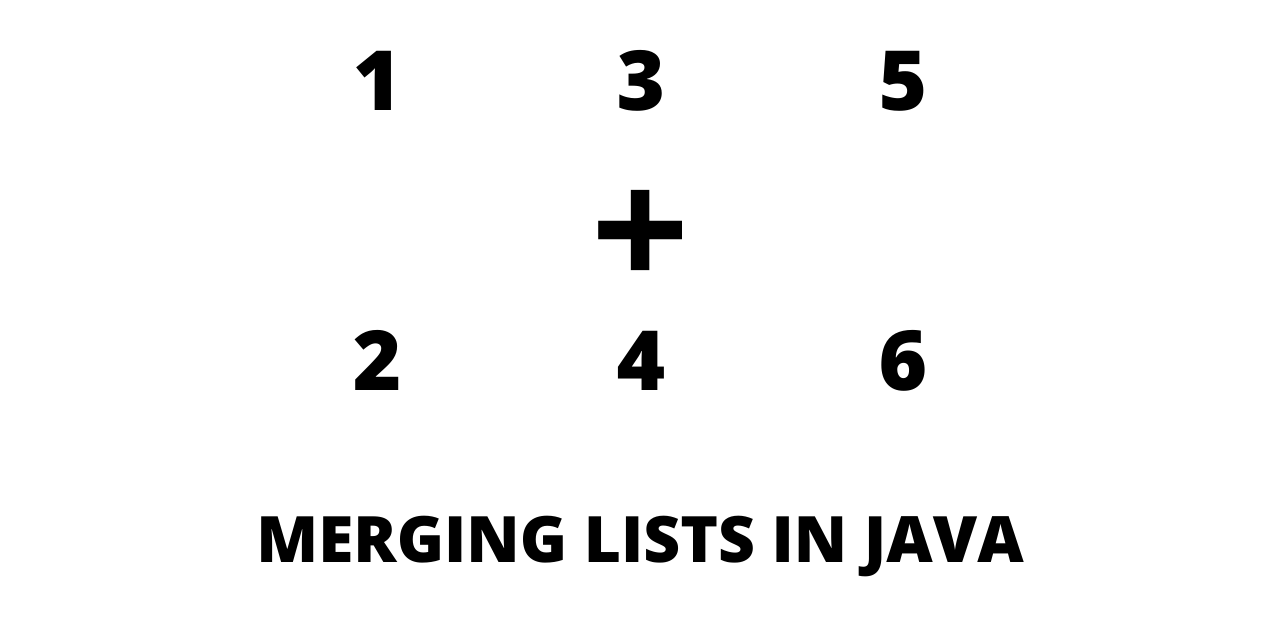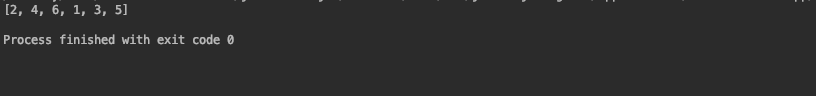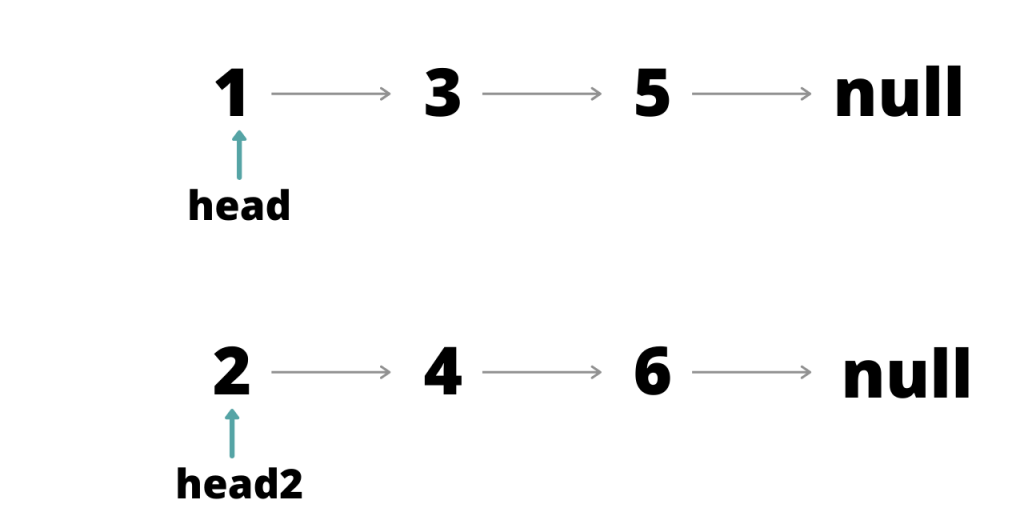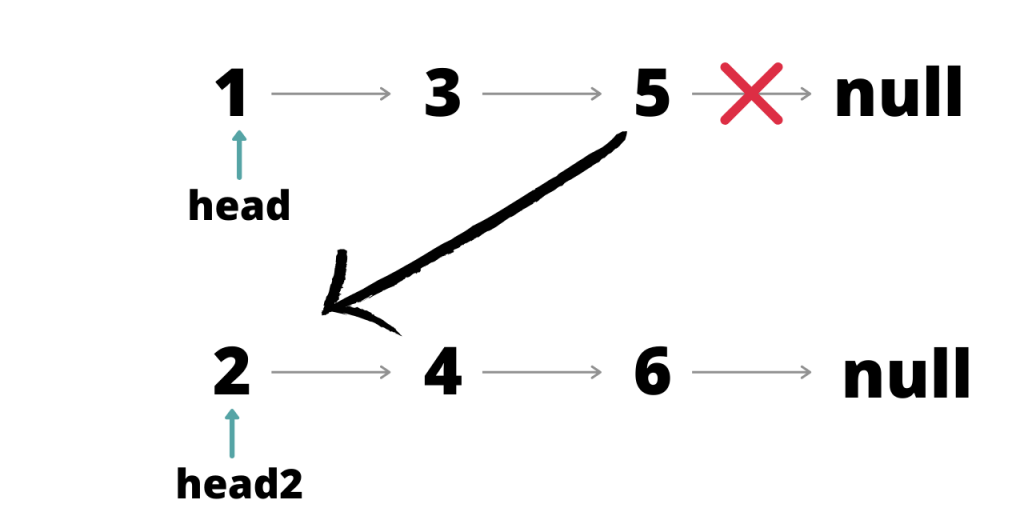# How to Merge Two Lists in Java?

Published on August 3, 2022By Jayant VermaWhile we believe that this content benefits our community, we have not yet thoroughly reviewed it. If you have any suggestions for improvements, please let us know by clicking the “report an issue“ button at the bottom of the tutorial.

Merging two lists in Java is often a useful operation. These lists can be ArrayLists or LinkedLists.

## How to Merge Two Lists in Java

There are multiple ways we can merge two lists in Java. Let’s explore some of the straightforward ones to get your job done!

### 1. The addAll() method to merge two lists

The addAll() method is the simplest and most common way to merge two lists.

For ArrayList :

``````import java.util.ArrayList;

public class Main {

public static void main(String[] args)
{
ArrayList<Integer> l1 = new ArrayList<Integer>();
ArrayList<Integer> l2 = new ArrayList<Integer>();
ArrayList<Integer> merge = new ArrayList<Integer>();
System.out.println("L1 : "+l1);
System.out.println("L2 : "+l2);
System.out.println("Merged : "+merge);
}
}
``````

Note the order of appearance of elements matches the order in which addAll() is called.

``````import java.util.LinkedList;

public class Main {

public static void main(String[] args)
{

System.out.println("L1 : "+L1);
System.out.println("L2 : "+L2);
System.out.println("Merged : "+merged);
}
}
``````

### 2. Using iterators to merge two lists in Java

We can use an Iterator to traverse the list and merge.

For ArrayList :

``````import java.util.ArrayList;

public class Main {

public static void main(String[] args)
{
ArrayList<Integer> l1 = new ArrayList<Integer>();
ArrayList<Integer> l2 = new ArrayList<Integer>();

ArrayList<Integer> Itmerge = new ArrayList<>();
Iterator i = l1.iterator();
while (i.hasNext()) {
}
i=l2.iterator();
while (i.hasNext()) {
}
System.out.println("L1 : "+l1);
System.out.println("L2 : "+l2);
System.out.println("Merged : "+Itmerge);
}
}
``````

Iterator first traverses the ArrayList l1 and adds all the elements to Itmerge, it then traverses the ArrayList l2 and adds all the elements to Itmerge.

Another way to merge the two lists is to simply add elements from one list to the other. There is no need to create a new list unless you need to keep the existing data intact.

``````Iterator i = l1.iterator();
while (i.hasNext())
{
}

System.out.println("Merged : "+l2);
``````

In this case, all the elements are added to the list l2. This saves the memory spent in the creation of an extra list. Adding the elements of one list to another saves the extra traversal.

``````import java.util.LinkedList;

public class Main {

public static void main(String[] args)
{

Iterator i = L1.iterator();
while (i.hasNext()) {
}
System.out.println(L2);
}
}
``````### 3. Merge Multiple Lists using for loop

For loop is also useful to merge two lists.

For ArrayList:

``````import java.util.ArrayList;

public class Main {

public static void main(String[] args)
{
ArrayList<Integer> l1 = new ArrayList<Integer>();
ArrayList<Integer> l2 = new ArrayList<Integer>();
ArrayList<Integer> Itmerge = new ArrayList<>();
for(int i=0;i<l1.size();i++){
}
for(int i=0;i<l2.size();i++){
}
System.out.println(Itmerge);
}
}
``````

The loop traverses both the ArrayLists and adds each element one by one to a newly created list.

Adding the elements of one list to another saves the extra traversal.

`````` for(int i=0;i<l2.size();i++){
}
System.out.println(l1);
``````

This for loop adds the elements of l2 to l1 one by one. In this case, l1 will contain the final list of merged elements.

To understand the traversal of linked lists a little better let us define our own linked list.

This will require a class for nodes. A node needs two things, data and the address of the next node.

Code for class node :

``````public class node {
int data;
node next;
public node(int data){
this.data=data;
next=null;
}
}
``````

Note that the next is of type node since it stores the address of a node. Creating the same two lists as used in earlier examples:

``````public class Main {

public static void main(String[] args)
{
node temp = new node(3);
node temp1 = new node(5);
temp.next=temp1;
node temp2 = new node(4);
node temp3 = new node(6);
temp2.next=temp3;
}
}
``````

This will create lists that look like :Each arrow represents the next link. To link the two lists together we need to link the end of one list to the head of the second.This can be done as follows:

``````node trav=head;
while(trav.next!=null){
trav=trav.next;
}
``````

A node ‘trav’ is initiated and pointed at the head of the first list. The first list is traversed until trav reaches the end of the first list.

When the end is reached, it changes the next link of the last node to head of the second list. This forms a link between the two lists.

Printing all the lists:

``````public class Main {

public static void main(String[] args)
{
node temp = new node(3);
node temp1 = new node(5);
temp.next=temp1;
node temp2 = new node(4);
node temp3 = new node(6);
temp2.next=temp3;

//printing list 1
System.out.println("List 1 :");

while(trav!=null){
System.out.print(trav.data + " ");
trav=trav.next;
}

System.out.println();
//prinitng list 2
System.out.println("List 2 :");

while(trav!=null){
System.out.print(trav.data + " ");
trav=trav.next;
}
System.out.println();

//merging the two list

while(trav.next!=null){
trav=trav.next;
}
// printing merged list
System.out.println("merged list :");
while(trav!=null){
System.out.print(trav.data + " ");
trav=trav.next;
}
}
}
``````

## Conclusion

We saw the different ways to merge two lists in Java. These are ranged from inbuilt functions to basic for loops. The last example above gives a deeper understanding of how lists work in Java. Using the approach of the last example you can have more control over the order in which elements appear in the list.

Thanks for learning with the DigitalOcean Community. Check out our offerings for compute, storage, networking, and managed databases.Jayant Verma

author

#### Still looking for an answer?

Ask a questionSearch for more help

Click below to sign up and get \$200 of credit to try our products over 60 days!

### Popular Topics##### Hollie's Hub for Good

Working on improving health and education, reducing inequality, and spurring economic growth? We’d like to help.##### Become a contributor

You get paid; we donate to tech nonprofits.

##### Welcome to the developer cloud

DigitalOcean makes it simple to launch in the cloud and scale up as you grow – whether you’re running one virtual machine or ten thousand.© 2023 DigitalOcean, LLC.